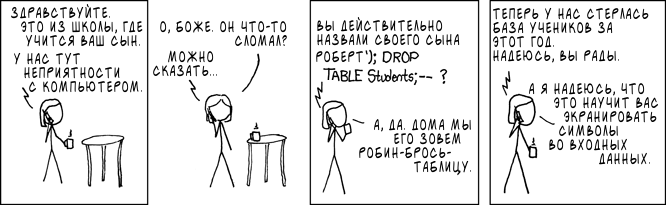0 like 0 dislike
40 views
Hi all, please tell me, what is wrong with the SQL query
``if (isset(\$_POST['date_btn'])) { \$surname = \$_POST['surname']; \$name = \$_POST['name']; \$patronymic = \$_POST['patronymic']; \$birth_date = \$_POST['birth_date']; \$e_mail = \$_POST['e-mail']; \$phone_number = \$_POST['phone_number']; \$region = \$_POST['region']; \$city = \$_POST['city']; \$school = \$_POST['school']; \$work_position = \$_POST['work_position']; mysqli_query(\$connect, "UPDATE users SET surname = '\$surname', name = '\$name', patronymic = '\$patronymic', birth_date = '\$birth_date', e_mail = '\$e_mail' phone_number = '\$phone_number' region = '\$region', city = '\$city', school = '\$school' work_position = '\$work_position' WHERE login = '\$login';"); header('Location: https://********.**/?page=date'); }``
| 40 views

0 like 0 dislike
commas lacking
``e_mail = '\$e_mail' phone_number = '\$phone_number' region``

must be
``e_mail = '\$e_mail', phone_number = '\$phone_number', region``by

0 like 0 dislike